Скачать презентацию Binary Numbers Numbering Systems Counting

89dce16f1acd0d147854c6618aeaca4f.ppt

• Количество слайдов: 25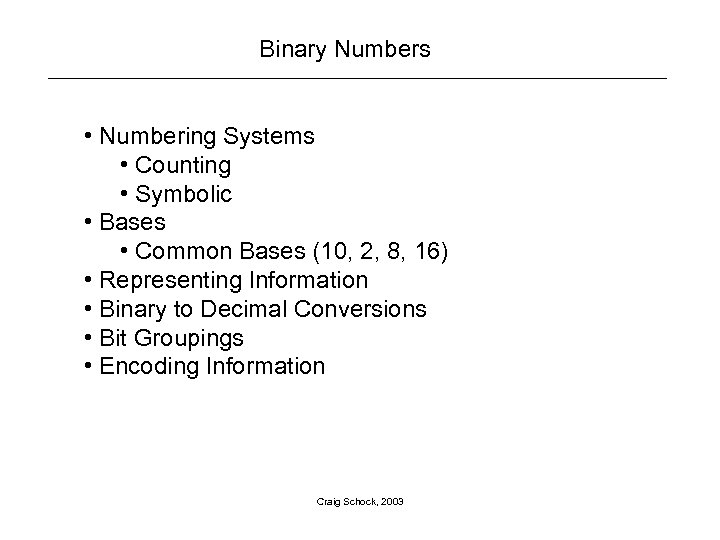Binary Numbers • Numbering Systems • Counting • Symbolic • Bases • Common Bases (10, 2, 8, 16) • Representing Information • Binary to Decimal Conversions • Bit Groupings • Encoding Information Craig Schock, 2003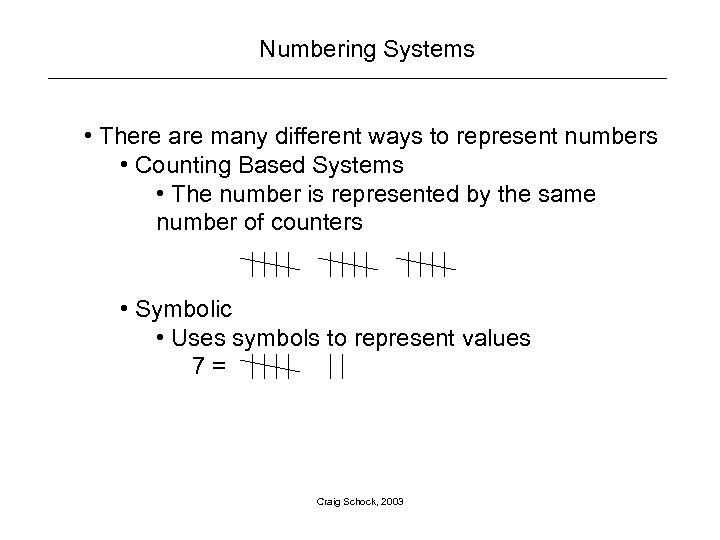Numbering Systems • There are many different ways to represent numbers • Counting Based Systems • The number is represented by the same number of counters • Symbolic • Uses symbols to represent values 7= Craig Schock, 2003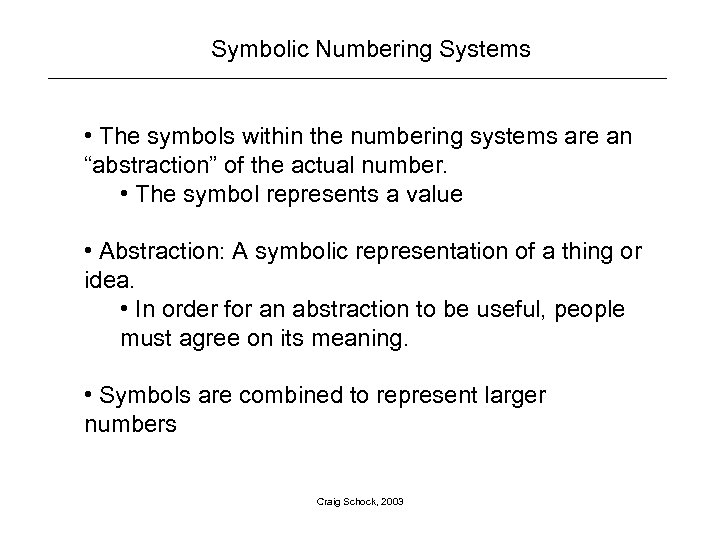Symbolic Numbering Systems • The symbols within the numbering systems are an “abstraction” of the actual number. • The symbol represents a value • Abstraction: A symbolic representation of a thing or idea. • In order for an abstraction to be useful, people must agree on its meaning. • Symbols are combined to represent larger numbers Craig Schock, 2003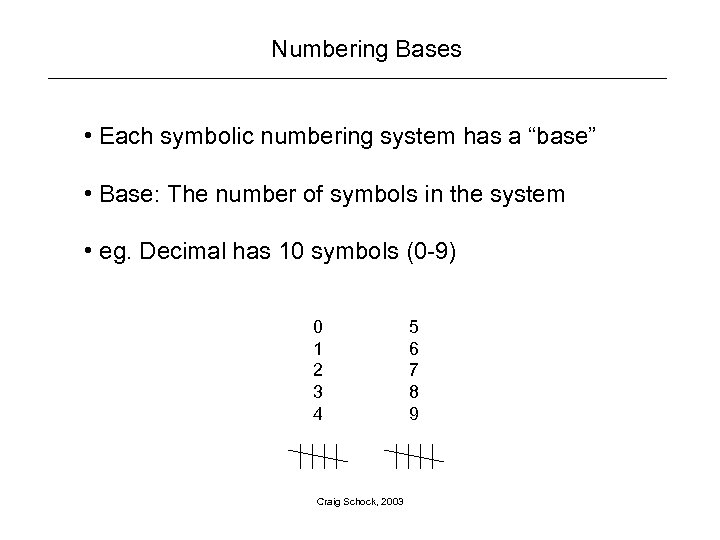Numbering Bases • Each symbolic numbering system has a “base” • Base: The number of symbols in the system • eg. Decimal has 10 symbols (0 -9) 0 1 2 3 4 Craig Schock, 2003 5 6 7 8 9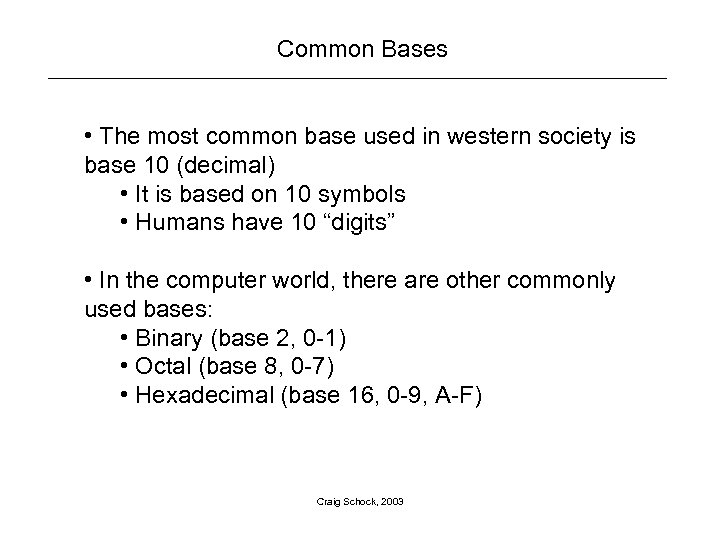Common Bases • The most common base used in western society is base 10 (decimal) • It is based on 10 symbols • Humans have 10 “digits” • In the computer world, there are other commonly used bases: • Binary (base 2, 0 -1) • Octal (base 8, 0 -7) • Hexadecimal (base 16, 0 -9, A-F) Craig Schock, 2003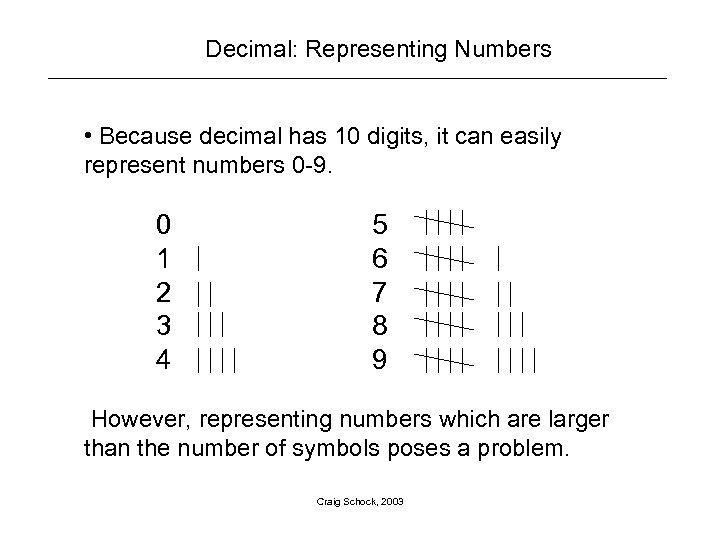Decimal: Representing Numbers • Because decimal has 10 digits, it can easily represent numbers 0 -9. 0 1 2 3 4 5 6 7 8 9 However, representing numbers which are larger than the number of symbols poses a problem. Craig Schock, 2003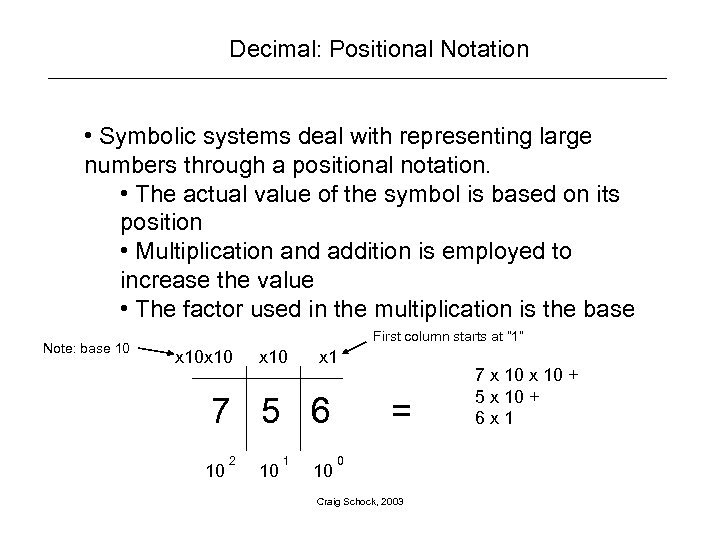Decimal: Positional Notation • Symbolic systems deal with representing large numbers through a positional notation. • The actual value of the symbol is based on its position • Multiplication and addition is employed to increase the value • The factor used in the multiplication is the base Note: base 10 First column starts at “ 1” x 10 x 1 7 5 6 10 2 10 1 10 = 0 Craig Schock, 2003 7 x 10 + 5 x 10 + 6 x 1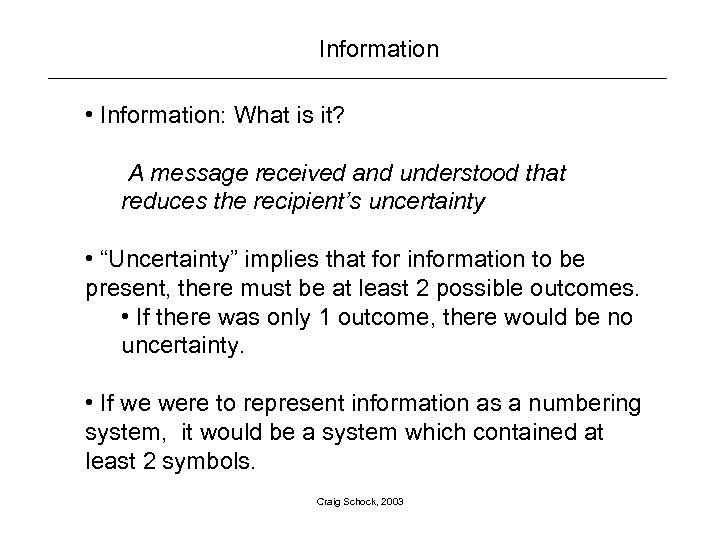Information • Information: What is it? A message received and understood that reduces the recipient’s uncertainty • “Uncertainty” implies that for information to be present, there must be at least 2 possible outcomes. • If there was only 1 outcome, there would be no uncertainty. • If we were to represent information as a numbering system, it would be a system which contained at least 2 symbols. Craig Schock, 2003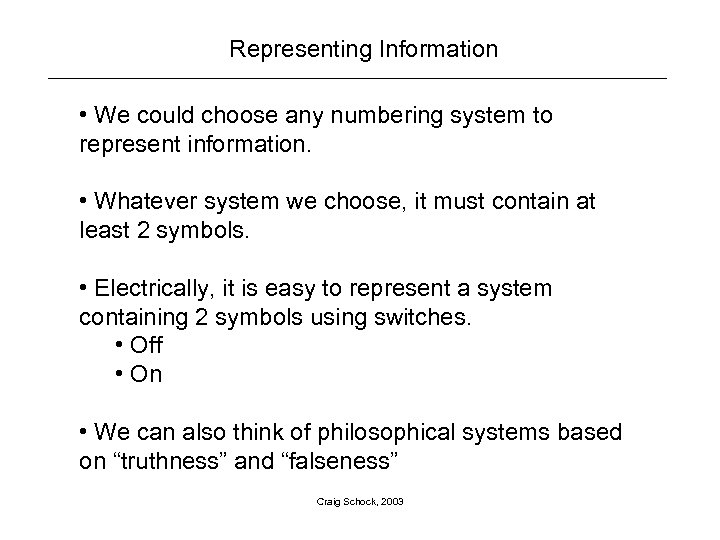Representing Information • We could choose any numbering system to represent information. • Whatever system we choose, it must contain at least 2 symbols. • Electrically, it is easy to represent a system containing 2 symbols using switches. • Off • On • We can also think of philosophical systems based on “truthness” and “falseness” Craig Schock, 2003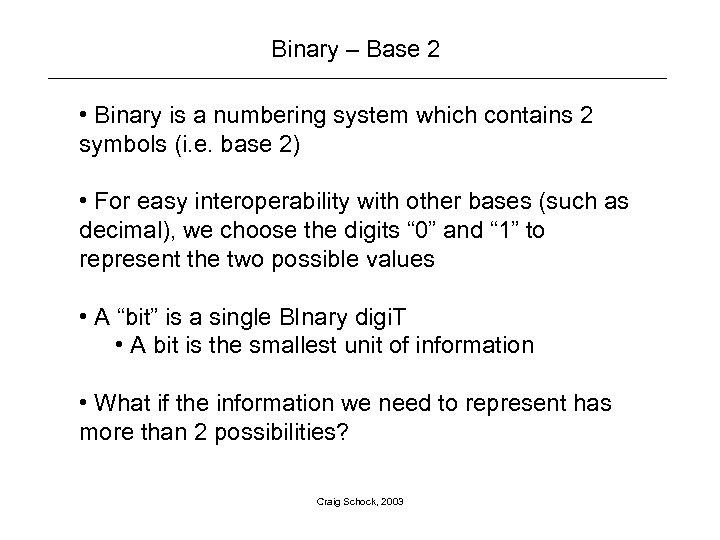Binary – Base 2 • Binary is a numbering system which contains 2 symbols (i. e. base 2) • For easy interoperability with other bases (such as decimal), we choose the digits “ 0” and “ 1” to represent the two possible values • A “bit” is a single BInary digi. T • A bit is the smallest unit of information • What if the information we need to represent has more than 2 possibilities? Craig Schock, 2003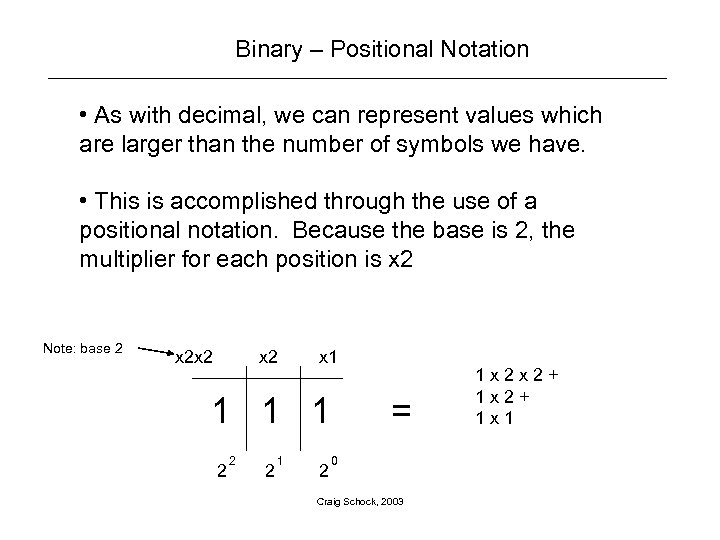Binary – Positional Notation • As with decimal, we can represent values which are larger than the number of symbols we have. • This is accomplished through the use of a positional notation. Because the base is 2, the multiplier for each position is x 2 Note: base 2 x 2 x 2 x 1 1 2 2 2 1 2 = 0 Craig Schock, 2003 1 x 2 x 2+ 1 x 1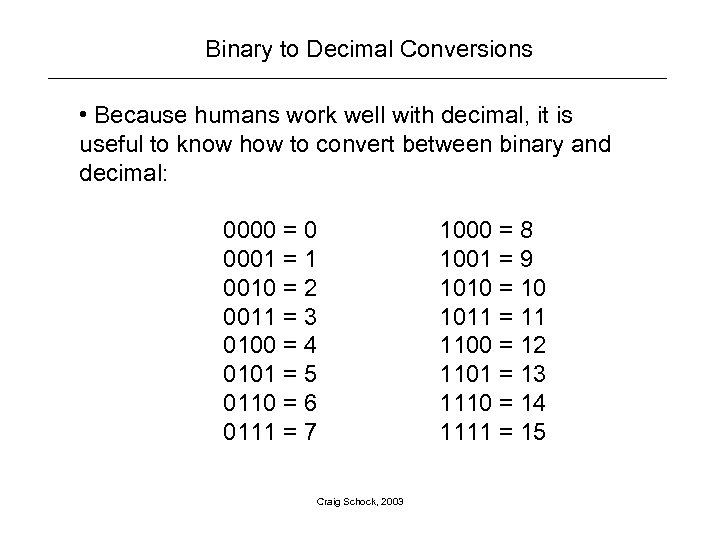Binary to Decimal Conversions • Because humans work well with decimal, it is useful to know how to convert between binary and decimal: 0000 = 0 0001 = 1 0010 = 2 0011 = 3 0100 = 4 0101 = 5 0110 = 6 0111 = 7 Craig Schock, 2003 1000 = 8 1001 = 9 1010 = 10 1011 = 11 1100 = 12 1101 = 13 1110 = 14 1111 = 15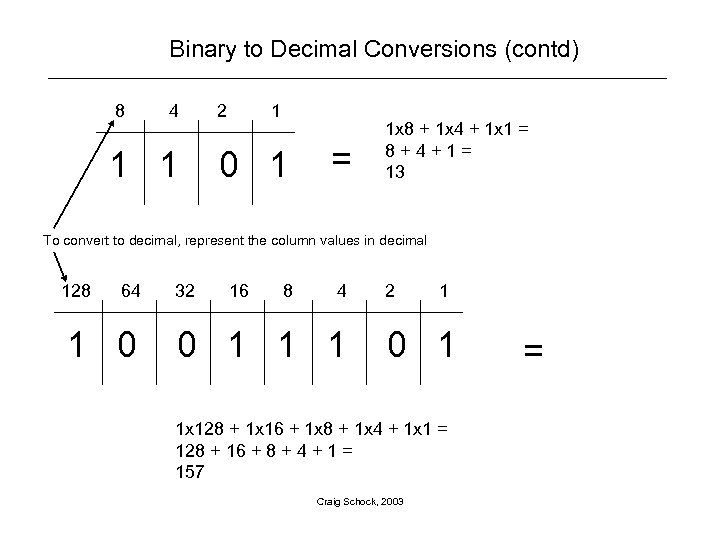Binary to Decimal Conversions (contd) 8 4 1 1 2 1 0 1 = 1 x 8 + 1 x 4 + 1 x 1 = 8+4+1= 13 To convert to decimal, represent the column values in decimal 128 64 1 0 32 16 8 4 0 1 1 1 2 1 0 1 1 x 128 + 1 x 16 + 1 x 8 + 1 x 4 + 1 x 1 = 128 + 16 + 8 + 4 + 1 = 157 Craig Schock, 2003 =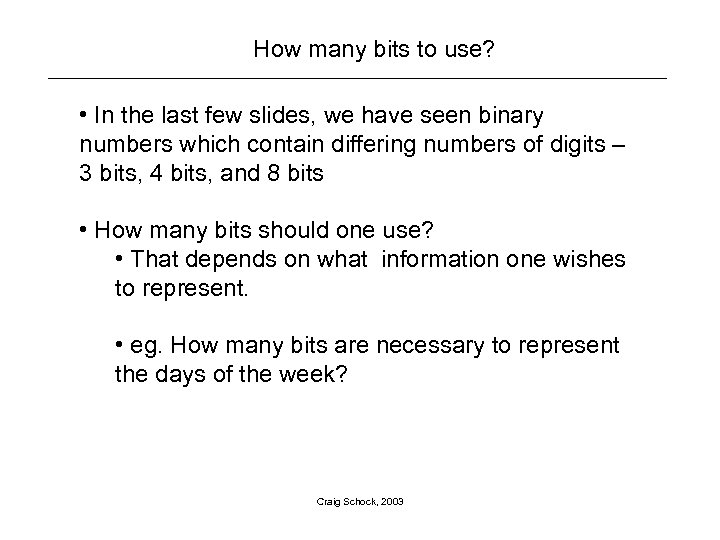How many bits to use? • In the last few slides, we have seen binary numbers which contain differing numbers of digits – 3 bits, 4 bits, and 8 bits • How many bits should one use? • That depends on what information one wishes to represent. • eg. How many bits are necessary to represent the days of the week? Craig Schock, 2003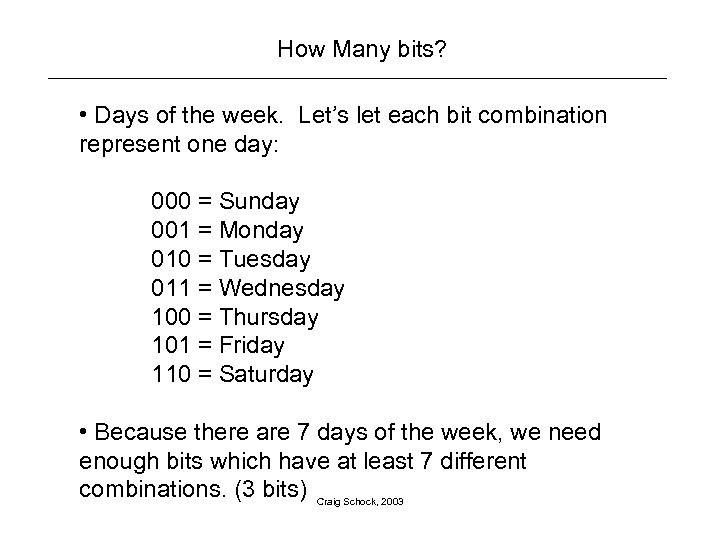How Many bits? • Days of the week. Let’s let each bit combination represent one day: 000 = Sunday 001 = Monday 010 = Tuesday 011 = Wednesday 100 = Thursday 101 = Friday 110 = Saturday • Because there are 7 days of the week, we need enough bits which have at least 7 different combinations. (3 bits) Craig Schock, 2003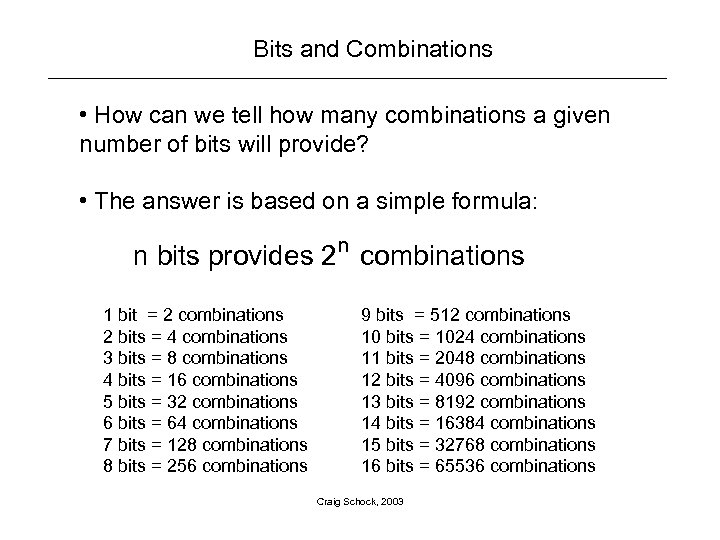Bits and Combinations • How can we tell how many combinations a given number of bits will provide? • The answer is based on a simple formula: n n bits provides 2 combinations 1 bit = 2 combinations 2 bits = 4 combinations 3 bits = 8 combinations 4 bits = 16 combinations 5 bits = 32 combinations 6 bits = 64 combinations 7 bits = 128 combinations 8 bits = 256 combinations 9 bits = 512 combinations 10 bits = 1024 combinations 11 bits = 2048 combinations 12 bits = 4096 combinations 13 bits = 8192 combinations 14 bits = 16384 combinations 15 bits = 32768 combinations 16 bits = 65536 combinations Craig Schock, 2003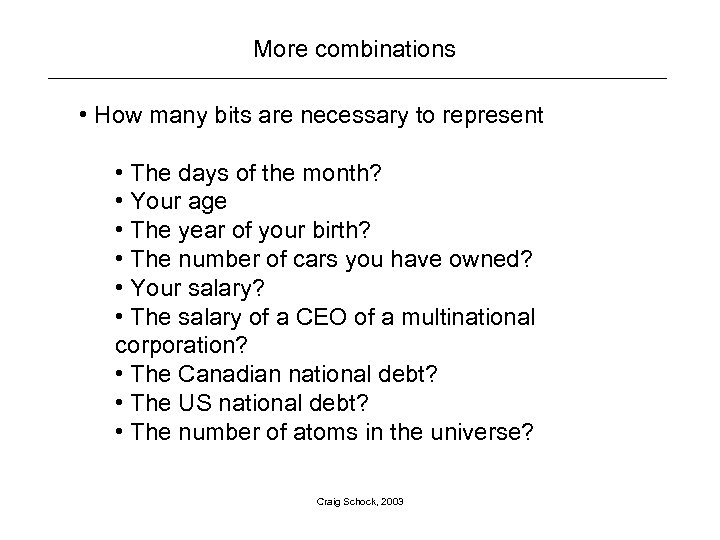More combinations • How many bits are necessary to represent • The days of the month? • Your age • The year of your birth? • The number of cars you have owned? • Your salary? • The salary of a CEO of a multinational corporation? • The Canadian national debt? • The US national debt? • The number of atoms in the universe? Craig Schock, 2003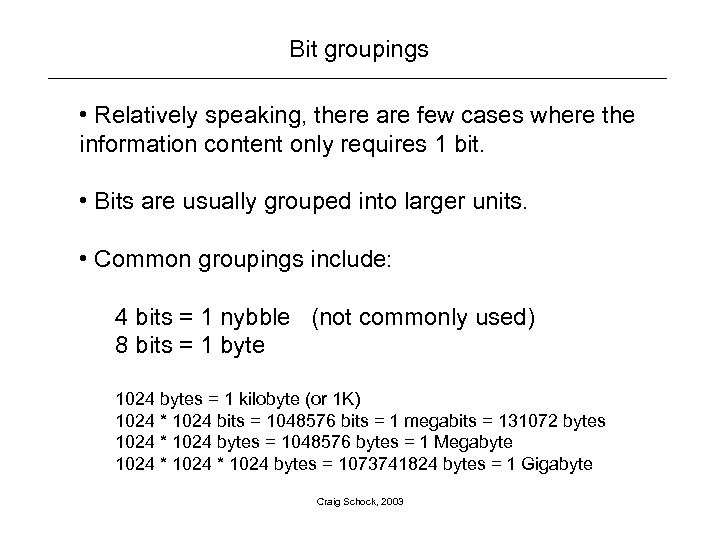Bit groupings • Relatively speaking, there are few cases where the information content only requires 1 bit. • Bits are usually grouped into larger units. • Common groupings include: 4 bits = 1 nybble (not commonly used) 8 bits = 1 byte 1024 bytes = 1 kilobyte (or 1 K) 1024 * 1024 bits = 1048576 bits = 1 megabits = 131072 bytes 1024 * 1024 bytes = 1048576 bytes = 1 Megabyte 1024 * 1024 bytes = 1073741824 bytes = 1 Gigabyte Craig Schock, 2003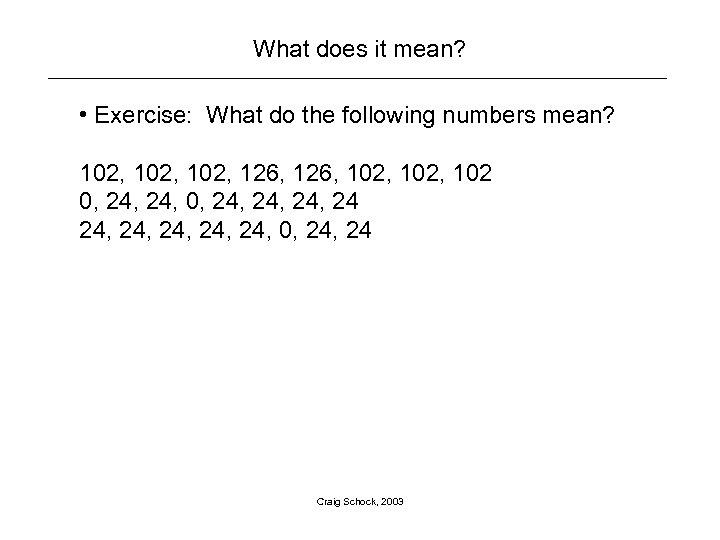What does it mean? • Exercise: What do the following numbers mean? 102, 126, 102, 102 0, 24, 24, 24, 0, 24 Craig Schock, 2003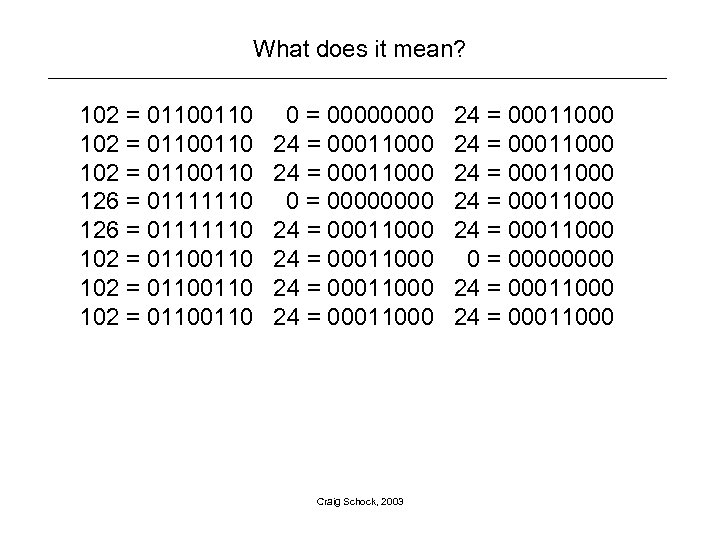What does it mean? 102 = 01100110 102 = 0110 126 = 01111110 102 = 01100110 102 = 0110 0 = 00000000 24 = 00011000 24 = 00011000 Craig Schock, 2003 24 = 00011000 24 = 00011000 0 = 0000 24 = 00011000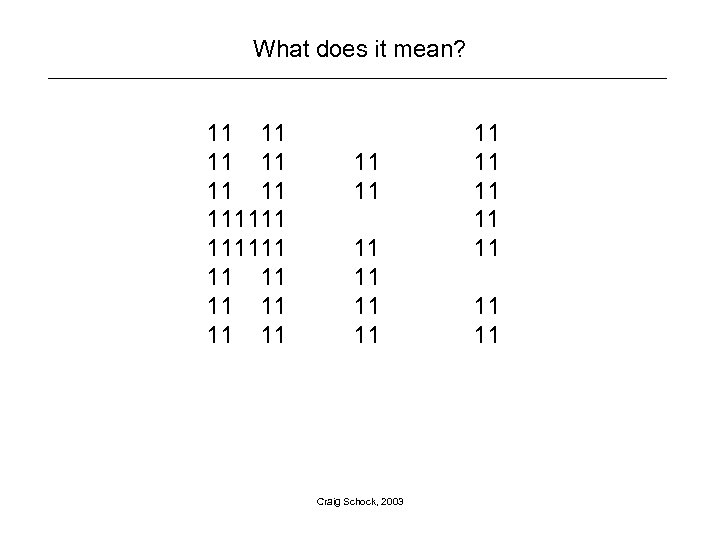What does it mean? 11 111111 11 11 11 Craig Schock, 2003 11 11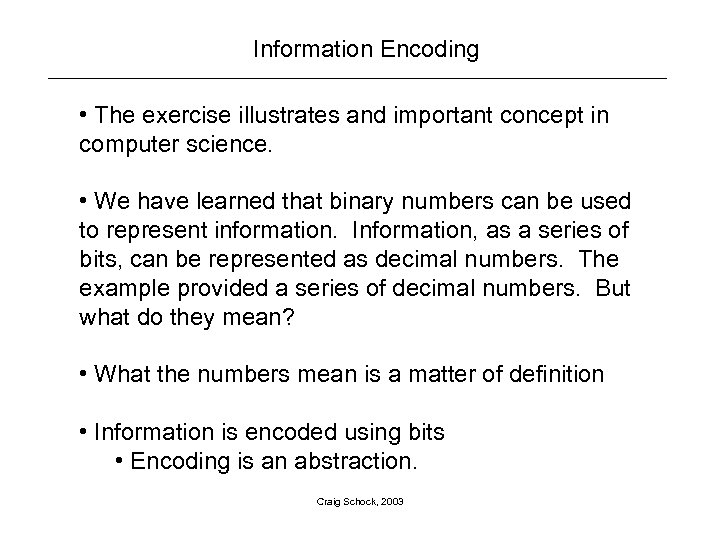Information Encoding • The exercise illustrates and important concept in computer science. • We have learned that binary numbers can be used to represent information. Information, as a series of bits, can be represented as decimal numbers. The example provided a series of decimal numbers. But what do they mean? • What the numbers mean is a matter of definition • Information is encoded using bits • Encoding is an abstraction. Craig Schock, 2003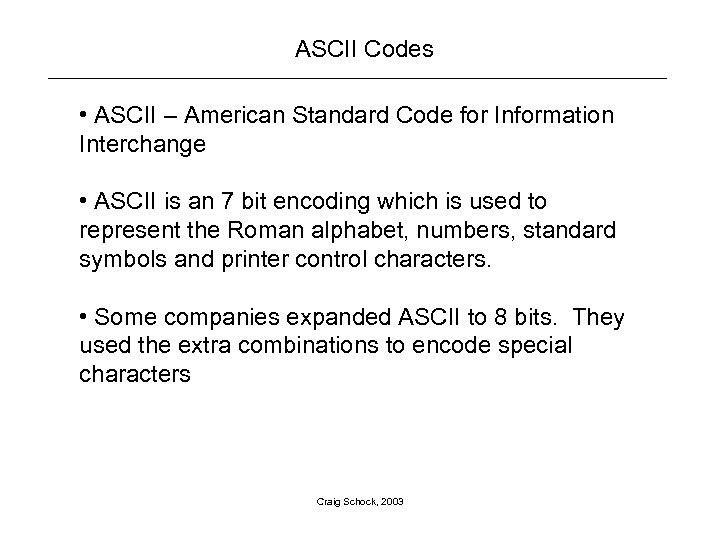ASCII Codes • ASCII – American Standard Code for Information Interchange • ASCII is an 7 bit encoding which is used to represent the Roman alphabet, numbers, standard symbols and printer control characters. • Some companies expanded ASCII to 8 bits. They used the extra combinations to encode special characters Craig Schock, 2003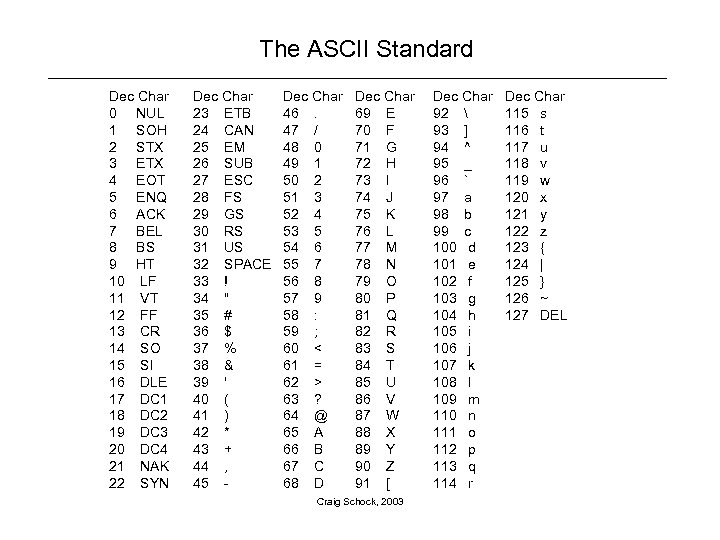The ASCII Standard Dec Char 0 NUL 1 SOH 2 STX 3 ETX 4 EOT 5 ENQ 6 ACK 7 BEL 8 BS 9 HT 10 LF 11 VT 12 FF 13 CR 14 SO 15 SI 16 DLE 17 DC 1 18 DC 2 19 DC 3 20 DC 4 21 NAK 22 SYN Dec Char 23 ETB 24 CAN 25 EM 26 SUB 27 ESC 28 FS 29 GS 30 RS 31 US 32 SPACE 33 ! 34 " 35 # 36 \$ 37 % 38 & 39 ' 40 ( 41 ) 42 * 43 + 44 , 45 - Dec Char 46. 47 / 48 0 49 1 50 2 51 3 52 4 53 5 54 6 55 7 56 8 57 9 58 : 59 ; 60 < 61 = 62 > 63 ? 64 @ 65 A 66 B 67 C 68 D Dec Char 69 E 70 F 71 G 72 H 73 I 74 J 75 K 76 L 77 M 78 N 79 O 80 P 81 Q 82 R 83 S 84 T 85 U 86 V 87 W 88 X 89 Y 90 Z 91 [ Craig Schock, 2003 Dec Char 92 93 ] 94 ^ 95 _ 96 ` 97 a 98 b 99 c 100 d 101 e 102 f 103 g 104 h 105 i 106 j 107 k 108 l 109 m 110 n 111 o 112 p 113 q 114 r Dec Char 115 s 116 t 117 u 118 v 119 w 120 x 121 y 122 z 123 { 124 | 125 } 126 ~ 127 DEL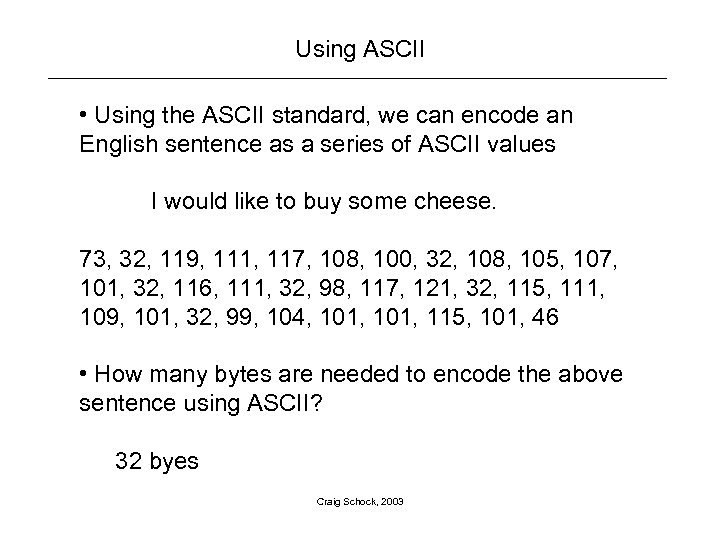Using ASCII • Using the ASCII standard, we can encode an English sentence as a series of ASCII values I would like to buy some cheese. 73, 32, 119, 111, 117, 108, 100, 32, 108, 105, 107, 101, 32, 116, 111, 32, 98, 117, 121, 32, 115, 111, 109, 101, 32, 99, 104, 101, 115, 101, 46 • How many bytes are needed to encode the above sentence using ASCII? 32 byes Craig Schock, 2003